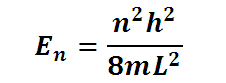# Energy of a particle in a 1-D box

This is a CalcTown calculator to calculate the energy of a particle in a 1-D box in its n-th excitation mode.

#### Result

J
meVwhere

me (given in the units) is the rest mass of an electron where me=9.10938291 × 10-31 kg

h= 6.62606957(29)×1034 J-s